# 人教版2021年八年级数学上册课时作业本 整式的乘除与因式分解-乘法公式（含答案）温馨提示：部分包含数学公式或PPT动画的文件，查看预览时可能会显示错乱或异常，文件下载后无此问题，请放心下载。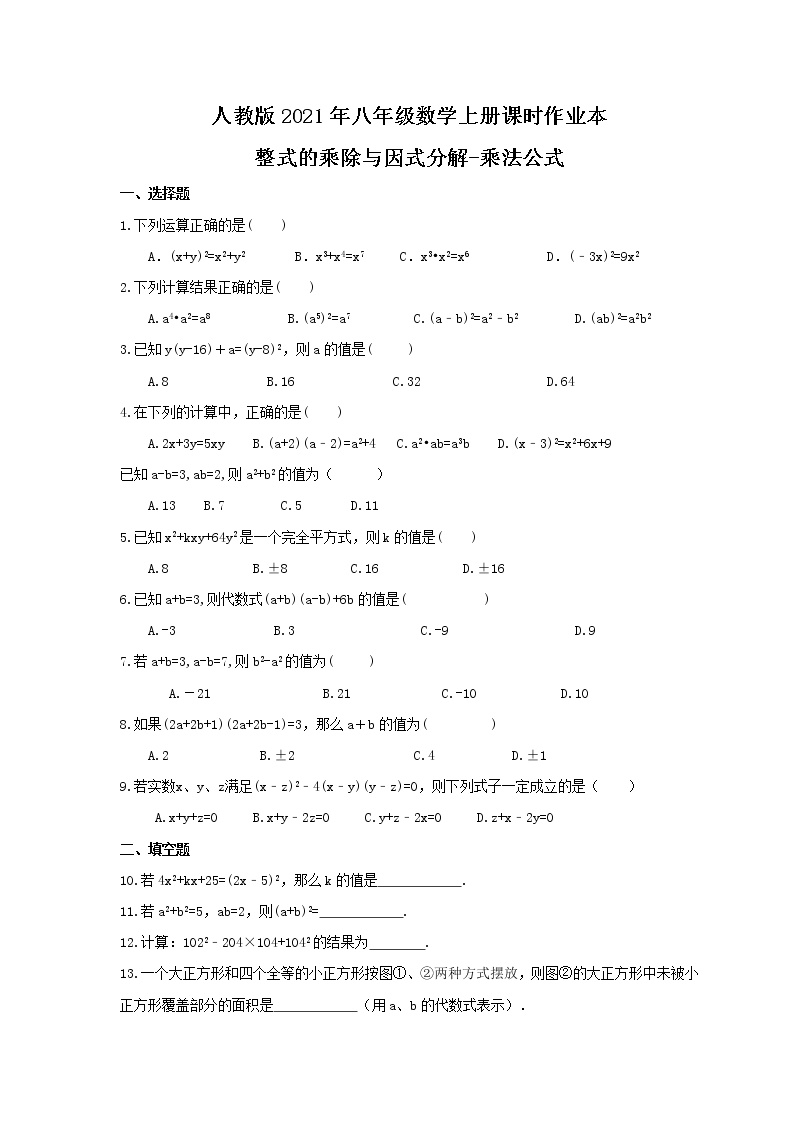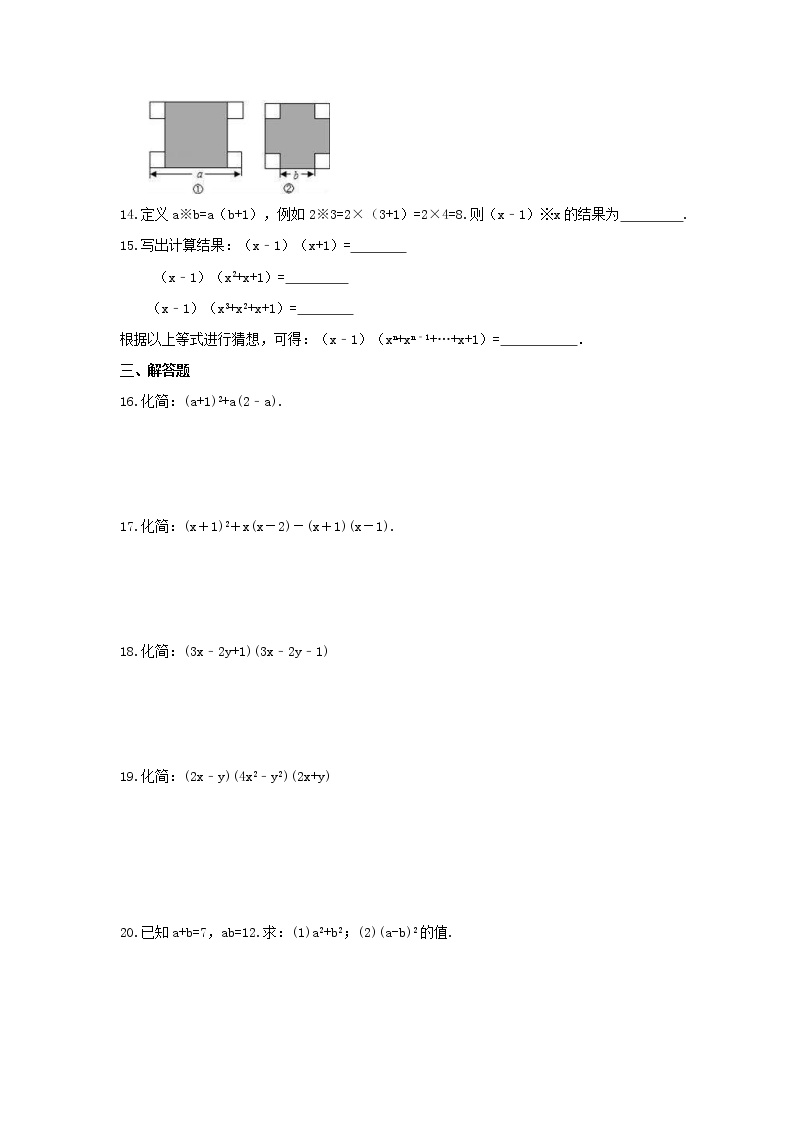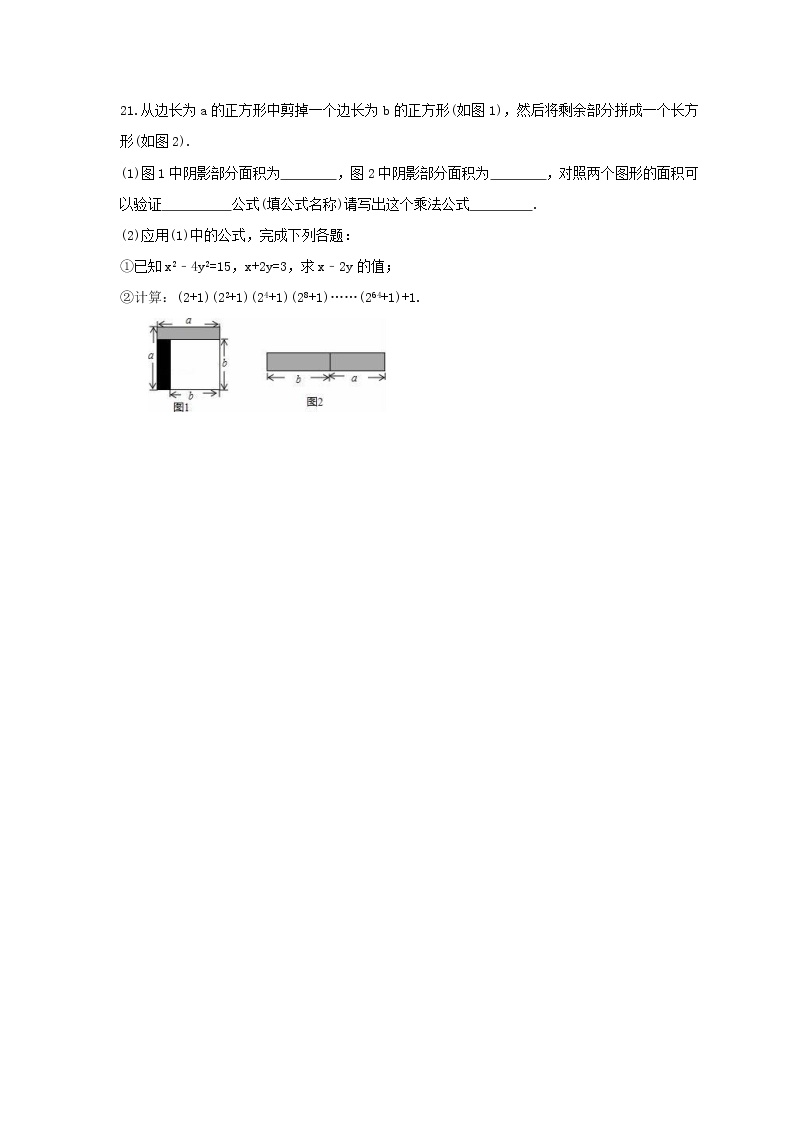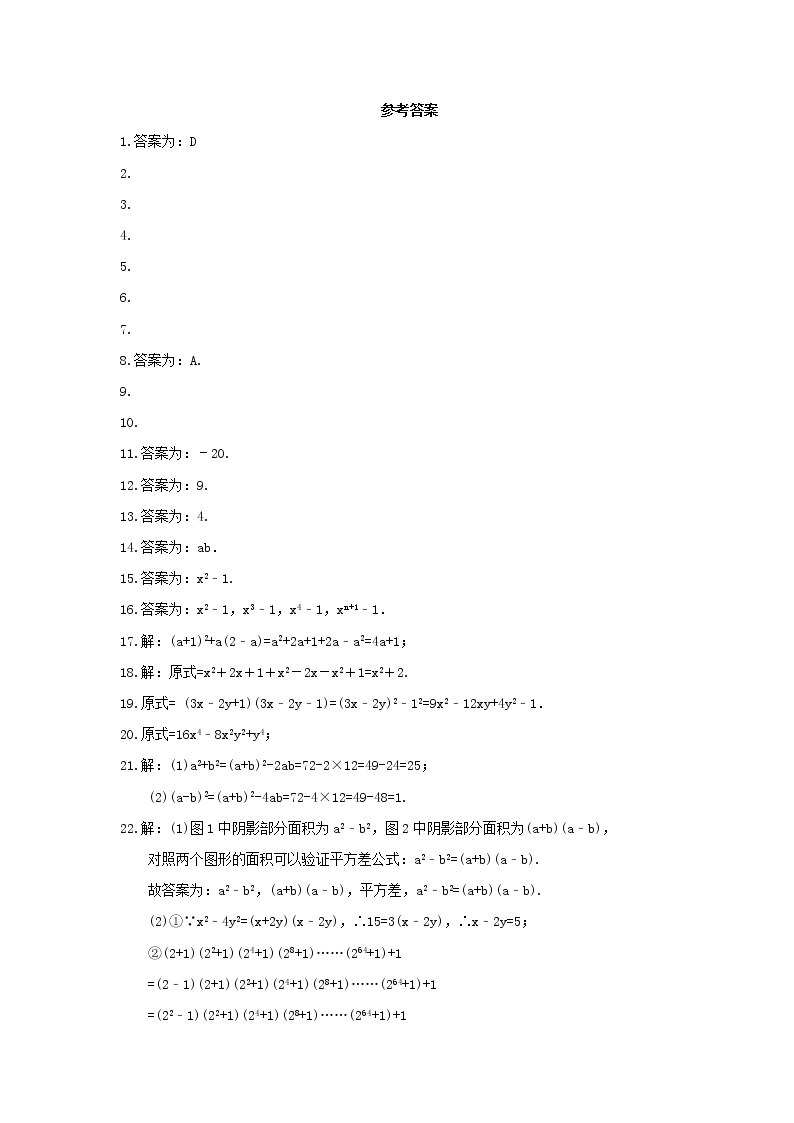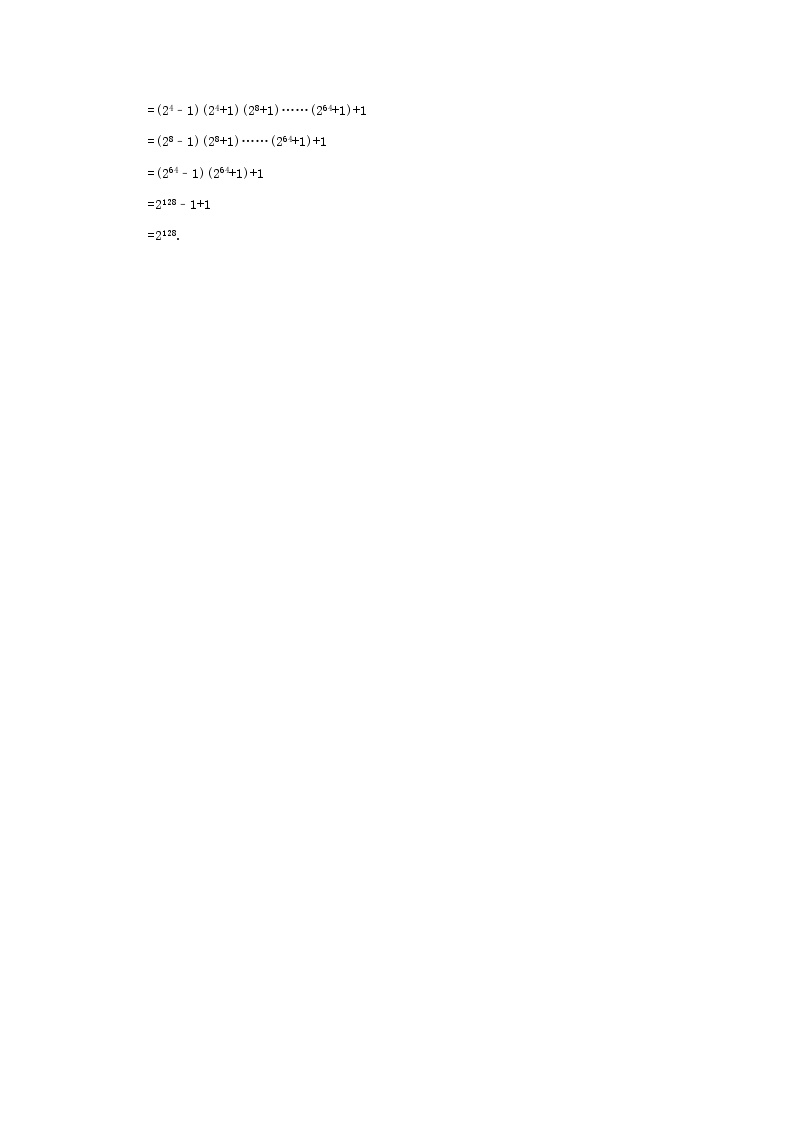、选择题

1.下列运算正确的是(　　)

A．(x+y)2=x2+y2    B．x3+x4=x7   C．x3x2=x6      D．(3x)2=9x2

2.下列计算结果正确的是(　　)

A.a4a2=a8          B.(a5)2=a7         C.(ab)2=a2b2      D.(ab)2=a2b2

3.已知y(y-16)＋a=(y-8)2，则a的值是(   )

A.8             B.16             C.32                 D.64

4.在下列的计算中，正确的是(　　)

A.2x+3y=5xy                  B.(a+2)(a2)=a2+4                 C.a2ab=a3b                  D.(x3)2=x2+6x+9

A.13                                B.7                                    C.5                     D.11

5.已知x2+kxy+64y2是一个完全平方式，则k的值是(　　)

A.8                          B.±8                       C.16                          D.±16

6.已知a+b=3,则代数式(a+b)(a-b)+6b的值是(     )

A.-3　　　     B.3　　　        C.-9　　       D.9

7.若a+b=3,a-b=7,则b2-a2的值为(   )

A.－21       B.21       C.-10      D.10

8.如果(2a+2b+1)(2a+2b-1)=3，那么a＋b的值为(     )

A.2      B.±2        C.4      D.±1

9.若实数x、y、z满足(xz)24(xy)(yz)=0，则下列式子一定成立的是（

A.x+y+z=0                   B.x+y2z=0                   C.y+z2x=0                   D.z+x2y=0

、填空题

10.若4x2+kx+25=(2x5)2，那么k的值是　　　　　　.

11.若a2+b2=5，ab=2，则(a+b)2=　　　　　　.

12.计算：1022204×104+1042的结果为        .

13.一个大正方形和四个全等的小正方形按图两种方式摆放，则图的大正方形中未被小正方形覆盖部分的面积是　　        （用a、b的代数式表示）．

14.定义ab=ab+1），例如23=2×（3+1）=2×4=8.则（x1）x的结果为　   　.

15.写出计算结果：（x1）（x+1）=

（x1）（x2+x+1）=

（x1）（x3+x2+x+1）=

、解答题

16.化简：(a+1)2+a(2a).

17.化简：(x＋1)2＋x(x－2)－(x＋1)(x－1).

18.化简：(3x2y+1)(3x2y1)

19.化简：(2xy)(4x2y2)(2x+y)

20.已知a+b=7，ab=12.求：(1)a2+b2；(2)(a-b)2的值.

21.从边长为a的正方形中剪掉一个边长为b的正方形(如图1)，然后将剩余部分拼成一个长方形(如图2).

(1)图1中阴影部分面积为　    　，图2中阴影部分面积为　    　，对照两个图形的面积可以验证　      　公式(填公式名称)请写出这个乘法公式　     　.

(2)应用(1)中的公式，完成下列各题：

1.答案为：D

2.D.

3.B

4.C

5.A

6.D

7.D

8.答案为：A.

9.D

10.D

11.答案为：20.

12.答案为：9.

13.答案为：4.

14.答案为：ab．

15.答案为：x21.

16.答案为：x21，x31，x41，xn+11．

17.解：(a+1)2+a(2a)=a2+2a+1+2aa2=4a+1；

18.解：原式=x2＋2x＋1＋x2－2x－x2＋1=x2＋2.

19.原式= (3x2y+1)(3x2y1)=(3x2y)212=9x212xy+4y21．

20.原式=16x48x2y2+y4

21.解：(1)a2+b2=(a+b)2-2ab=72-2×12=49-24=25；
(2)(a-b)2=(a+b)2-4ab=72-4×12=49-48=1.

22.解：(1)图1中阴影部分面积为a2b2，图2中阴影部分面积为(a+b)(ab)，

(2)①∵x24y2=(x+2y)(x2y)，15=3(x2y)，x2y=5；

(2+1)(22+1)(24+1)(28+1)……(264+1)+1

=(21)(2+1)(22+1)(24+1)(28+1)……(264+1)+1

=(221)(22+1)(24+1)(28+1)……(264+1)+1

=(241)(24+1)(28+1)……(264+1)+1

=(281)(28+1)……(264+1)+1

=(2641)(264+1)+1

=21281+1

=2128.• 充值下载
• 扫码直接下载
• 下载需要：0 学贝 账户剩余：0 学贝
学贝可用于下载教习网 400万 精选资源，300万 精选试题，在线组卷
• 想免费下载此资料？完善资料，立得50学贝
微信扫码注册微信扫码，快速注册注册可领 50 学贝

手机号注册注册可领 50 学贝
手机号码

手机号格式错误

手机验证码获取验证码

手机验证码已经成功发送，5分钟内有效

设置密码

6-20个字符，数字、字母或符号

注册即视为同意教习网「注册协议」「隐私条款」QQ注册手机号注册微信注册注册成功

#### 免费下载这份资料

当前资料价值0元

下载需支付0学贝

扫码邀请0名好友关注我们即可免费下载

微信扫一扫，将图片发送给好友

每邀请1人关注教习网即可获得5学贝，学贝秒到账，多邀多得无上限，在个人中心查看学贝明细

•注册有礼

•官方微信

官方
微信关注“教习网”公众号

打开微信就能找资料

•在线客服

在线
客服

QQ在线客服

在线咨询

周一至周五 8:30-20:30

电话客服

0755-23774055

周一至周五 8:30-16:30返回
顶部